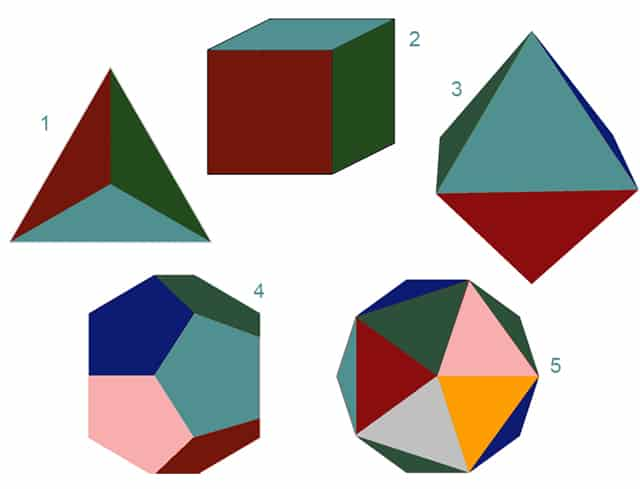# Interrelationships of the Past Crossed DisciplinesInterrelationships of the Past Crossed Disciplines. Numbers were an ancient reasoning tool.  Ancient philosophers and priests used common numbers to emphasize similarities. This blog simply illustrates one example: How Great Pyramid and the Platonic solids use the same numbers. I’ll walk my reader through the meaning of two diagrams.#1 = tetrahedron. #2 = cube. #3 = octahedron. #4 = dodecaedron. # 5 = icosahedron.

First: The dimensions of the Great Pyramid. Its essential dimensions are: Square base is 8 x 8 great cubits. The four triangulated faces peak together at 5 great cubits: Note-

• The base is square. It measures 440 shorter  Egyptian cubits of 1.71818..  feet on each side.
• One Great Cubit is 55 of such smaller cubits.
• The Great Pyramid measures 440 cubits or 8 such great cubits on each side.
• The truncated height is 275 of these smaller cubits.
• By great cubits this becomes 5.

Next we turn our attention to the 5 solids. First, 5 is the key number for both: The Great Pyramid has 5 faces. There are 5 Platonic solids.What is the source of  five? It is not an arbitrary number. Look at the 3 x 3 number square. Five is at its core. Very important: Interrelationships are deeper than they appear on this number square. Through opposite polarities they go to infinity! One example is in the next paragraph.

I mentioned each side of the Pyramid is 440 cubits. Where is 440 n this number square?  For this I must credit an Indian spirit I believe to be from the Lennie Lenape tribe. I spent many summers on Oquaga Lake.Oquaga Lake is where I worked as a professional pianist. I played many shows in the large, white building at Scott’s Oquaga Lake House. It is full of fun, wonderful memories and stories.

### Interrelationships Galore

Take the numbers around the perimeter. Add them two at the time as follows: 49 + 92 + 27 + 76 + 61 + 18 + 83 + 34 = 440. It is the same forwards or backwards. Each side of the square-based Great Pyramid is 440 cubits. It’s the numbers that are important: Ancients of varied civilizations attached their own units of measure to these key numbers. What was the primary reason the Great Pyramid was built?

1. To illustrate every way the 3 x 3 square can function.
2. With this knowledge, a new Golden Age of Peace and Plenty can be implemented

Next, look at the illustrations of the Platonic solids. Vertical column one (on the left) totals the features each solid.  Each of the five has its own horizontal column. This shows the added horizontal values. Here is the total number of faces, corners and edges for each solid. In other words, the topology.

• The tetrahedron has 14.
• The cube has 26.
• The octahedron has 26.
• The icosahedron has 62.
• The dodecahedron has 62.

This information can be found in standard textbooks on geometry. Next the chart reduces these topology numbers as follows:

• Fourteen: 1 +4 = 5. This is for the tetrahedron.
• Twenty-six: 2 + 6 = 8. This applies to the cube and octahedron.
• The third different number is sixty-two.  6 + 2 = 8. This applies to the dodecahedron and icosahedron.

We thus see four eights and one five. That duplicates the four-square base of 8 Great Cubits on each side and the pyramid’s height of 5 Great Cubits.The pot of gold at the end of the rainbow on Oquaga Lake holds knowledge.